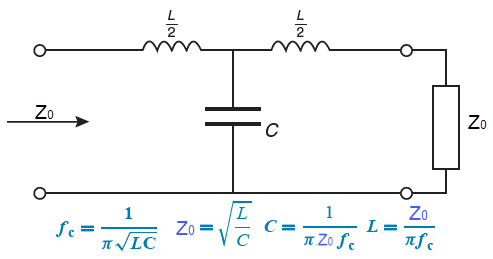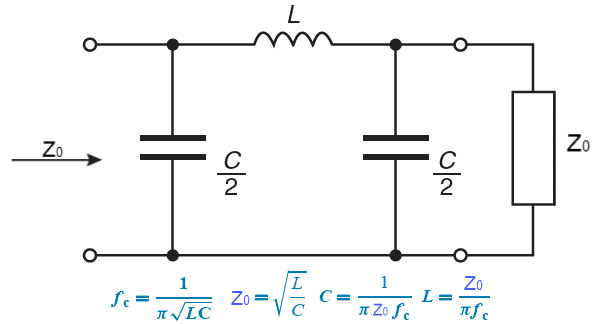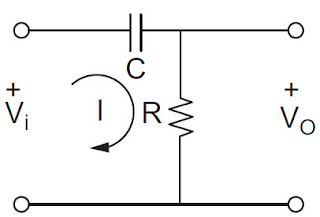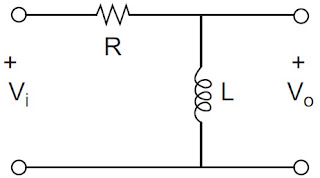# T and Pi LPF and HPF Calculator

T LPF Calculator
Calculate Frequency(fc):

Results:Calculate Zo:

Results:

Calculate L & C:

Results:

About T-network Low Pass Filter Online Calculator:
The T-network Low Pass Filter (LPF) online calculator is a user-friendly tool that allows engineers, hobbyists, and electronics enthusiasts to design and analyze T-network based low pass filters with ease. This online calculator is a valuable resource for anyone working on electronic circuits that require filtering of high-frequency signals and passing of low-frequency signals. The interface of the T-network LPF online calculator is simple and intuitive, making it accessible to users with varying levels of experience in electronics. Users can input the required parameters such as the cutoff frequency, the characteristic impedance of the transmission lines, and the desired filter order. The calculator then calculates the component values, including the inductance and capacitance values for the T-network, based on the user's input. The T-network LPF online calculator also provides a graphical representation of the filter response, allowing users to visualize the magnitude and phase of the filtered signal. This helps users to quickly assess the performance of the filter and make adjustments as needed. One of the notable features of the T-network LPF online calculator is its versatility. It supports various types of T-network configurations, including series-series (SS), shunt-shunt (SH), and series-shunt (SHP) configurations. This allows users to choose the most suitable T-network topology for their specific application requirements. Another advantage of the T-network LPF online calculator is that it provides flexibility in the choice of component values. Users can select commercially available inductors and capacitors or use custom component values, giving them the freedom to design filters that meet their specific needs. In summary, the T-network LPF online calculator is a powerful tool that simplifies the design process of T-network based low pass filters. With its user-friendly interface, graphical representation of filter response, support for various T-network configurations, and flexibility in component selection, it is a valuable resource for engineers and electronics enthusiasts involved in designing electronic circuits that require effective signal filtering.
T LPF Calculator
Calculate Frequency(fc):

Results:Calculate Zo:

Results:

Calculate L & C:

Results:

About PiT-network Low Pass Filter Online Calculator:
The Pi Network LPF (Linear Prediction Filter) online calculator is a powerful and user-friendly tool that allows users to accurately compute the LPF value for a given input signal. The calculator is specifically designed for use with the Pi Network, which is a mathematical algorithm used for digital signal processing applications. The LPF is an important element in digital signal processing, as it helps to filter out noise and unwanted frequencies from a signal, leaving behind the desired signal components. The Pi Network LPF is known for its efficiency and effectiveness in signal processing applications, making it a popular choice among engineers and researchers. The online calculator is easy to use, with a clean and intuitive interface. Users can input their signal data, such as the input signal frequency, cutoff frequency, and order of the filter. The calculator then employs the Pi Network algorithm to compute the LPF value, which represents the coefficients of the filter transfer function. The calculator provides detailed results, including the LPF coefficients and the transfer function equation. Users can also visualize the frequency response of the LPF, which allows for a better understanding of how the filter affects the input signal. Additionally, the calculator offers customization options, allowing users to adjust various parameters and experiment with different filter designs. This flexibility makes it a valuable tool for engineers, researchers, and students working on digital signal processing projects. Overall, the Pi Network LPF online calculator is a reliable and efficient tool for accurately calculating LPF coefficients for digital signal processing applications. Its user-friendly interface, detailed results, and customization options make it an invaluable resource for anyone involved in signal processing research or applications.
RC HPF Calculator
Calculate Frequency(fc):

Results:Calculate R(=XC):

Results:
Calculate C:

Results:

Formula:
Cutoff frequency:
$$f_0=\frac{1}{2 \pi R C}$$
Capactive Reactance:
$$X_C=\frac{1}{2 \pi f_0 C}$$
RL HPF Calculator
Calculate Frequency(fc):

Results:Calculate R(=XL):

Results:

Calculate L:

Results:

Formula:
Cutoff frequency:
$$f_0=\frac{R}{2 \pi L}$$
Inductive Reactance:
$$X_L=2 \pi f_0 L$$